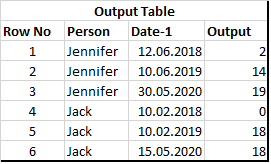# Calculating sum of quantities comparing dates of two tables

Hello,

I have the below input tables:For each person I want to apply the below procedure:

• Sum the quantity at the table-2, where the the date-2 is smaller than date-1

• For example, Jennifer has the date 10.06.2019 at row2 at the table-1. From table-2 find the rows which are less than 10.06.2019 and sum the all quantities at these rows. For that case, row 1 ,2 and 3 has smaller dates than 10.06.2019. So sum of these quantities are 14.

As a result the output table should be as below:Workflow is as below:

KNIME_create _table.knwf (6.5 KB)

I will be very glad for any help. Thanks a lot.

This should get you started:
https://hub.knime.com/elsamuel/spaces/Public/latest/KNIME_create_table

The values for Person and Date-1 from Table 1 are converted to variables and used for filtering. A GroupBy node calculates the sums.

3 Likes

@elsamuel, can you please share the work flow? I am stuck at the date filter node. Thanks.

I did share the workflow in my last response. It’s accessible and downloadable from my Hub space.

it is better to use Short Links when linking Hub workflows as this link is not supposed to change even if you change flow location to let’s say different space